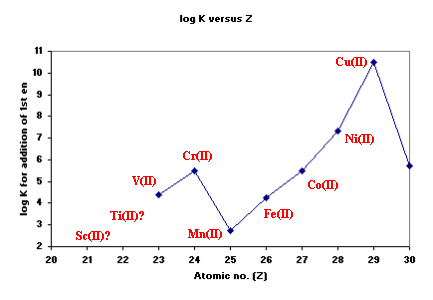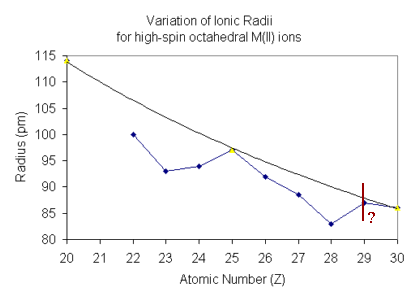The Irving-Williams series

The general stability sequence of high spin octahedral metal complexes for the replacement of water by other ligands is:

Mn(II) < Fe(II) < Co(II) < Ni(II) < Cu(II) > Zn(II)

This trend is essentially independent of the ligand.

In the case of 1,2-diaminoethane (en), the first step-wise stability constants (logK1) for M(II) ions are shown below.Notes
The sequence is generally quoted ONLY for Mn(II) to Zn(II) since there is little data available for the other first row transition metal ions; their M(II) oxidation states are not very stable.
The position of Cu(II) is considered out-of-line with predictions based on Crystal Field Theory and is probably a consequence of the fact that Cu(II) often forms distorted octahedral complexes. (See notes on the Jahn-Teller effect).

One explanation
Crystal Field Theory is based on the idea that a purely electrostatic interaction exists between the central metal ion and the ligands. This suggests that the stability of the complexes should be related to the ionic potential; that is, the charge to radius ratio. In the Irving-Williams series, the trend is based on high-spin M(II) ions, so what needs to be considered is how the ionic radii vary across the d-block.

For free metal ions in the gaseous phase it might be expected that the ionic radius of each ion on progressing across the d-block should show a gradual decrease in size. This would come about due to the incomplete screening of the additional positive charge by the additional electron, as is observed in the Lanthanide Contraction.
For high-spin octahedral complexes it is essential to consider the effect of the removal of the degeneracy of the d-orbitals by the crystal field. Here the d-electrons will initially add to the lower t2g orbitals before filling the eg orbitals since for octahedral complexes, the t2g subset are directed in between the incoming ligands whilst the eg subset are directed towards the incoming ligands and cause maximum repulsion.For d1-d3 (and d6-d8) the addition of the electrons to the t2g orbitals will mean that the screening of the increasing attractive nuclear charge is not very effective and the radius should be smaller than for the free ion.

The position of d4 and d9 on the plot is difficult to ascertain with certainty since six-coordinate complexes are expected to be distorted due to the Jahn-Teller Theorem. Cr(II) is not very stable so few measurements are available. For Cu(II) however, most complexes are found to have 4 short bonds and 2 long bonds although 2 short and 4 long bonds is feasible. The radii are expected to show an increase over the d3 and d8 situation since electrons are being added to the eg subset. The reported values have been found to lie on both sides of the predicted value.

For d0, d5 and d10 the screening expected is essentially that of a spherical arrangement equivalent to the absence of a crystal field. The plot above shows that these points return to the line drawn showing a gradual decrease of the radius on moving across the d-block.

Once the decrease in radius with Z pattern is understood, it is a small step to move to a pattern for q/r since this only involves taking the reciprocal of the radius and holding the charge constant. The radius essentially decreases with increasing Z, therefore 1/r must increase with increasing Z.

For the sequence Mn(II) to Zn(II), the crystal field (q/r) trend expected would be:

Mn(II) < Fe(II) < Co(II) < Ni(II) > Cu(II) > Zn(II)

Apart from the position of Cu(II), this corresponds to the Irving-Williams series. The discrepancy is once again accounted for by the fact that copper(II) complexes are often distorted or not octahedral at all. When this is taken into consideration, it is seen that the Irving-Williams series can be explained quite well using Crystal Field Theory.Return to Chemistry, UWI-Mona, Home Page